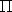## RegularTriangulationTraits_3

### Definition

The concept RegularTriangulationTraits_3 is the first template parameter of the class Regular_triangulation_3. It defines the geometric objects (points, segments...) forming the triangulation together with a few geometric predicates and constructions on these objects.

### Refines

TriangulationTraits_3

In addition to the requirements described for the traits class of Triangulation_3, the geometric traits class of Regular_triangulation_3 must fulfill the following requirements.

### Types

 RegularTriangulationTraits_3::Line_3 The line type. RegularTriangulationTraits_3::Object_3 The object type. RegularTriangulationTraits_3::Plane_3 The plane type. RegularTriangulationTraits_3::Ray_3 The ray type.

We use here the same notation as in Section 27.3. To simplify notation, p will often denote in the sequel either the point p3 or the weighted point p(w)=(p,wp).

RegularTriangulationTraits_3::Weighted_point_3
The weighted point type.

RegularTriangulationTraits_3::Power_test_3
A predicate object which must provide the following function operators:

Oriented_side operator()( Weighted_point_3 p, Weighted_point_3 q, Weighted_point_3 r, Weighted_point_3 s, Weighted_point_3 t),
which performs the following:
Let z(p,q,r,s)(w) be the power sphere of the weighted points (p,q,r,s). Returns
ON_ORIENTED_BOUNDARY if t is orthogonal to z(p,q,r,s)(w),
ON_NEGATIVE_SIDE if t lies outside the oriented sphere of center z(p,q,r,s) and radius sqrt( wz(p,q,r,s)2 + wt2 ) (which is equivalent to(t(w),z(p,q,r,s)(w) >0)),
ON_POSITIVE_SIDE if t lies inside this oriented sphere.
 Precondition: p, q, r, s are not coplanar. Note that with this definition, if all the points have a weight equal to 0, then power_test_3(p,q,r,s,t) = side_of_oriented_sphere(p,q,r,s,t). Oriented_side operator()( Weighted_point_3 p, Weighted_point_3 q, Weighted_point_3 r, Weighted_point_3 t), which has an definition analogous to the previous method, for coplanar points, with the power circle z(p,q,r)(w).
 Precondition: p, q, r are not collinear and p, q, r, t are coplanar. If all the points have a weight equal to 0, then power_test_3(p,q,r,s,t) = side_of_oriented_circle(p,q,r,s,t). Oriented_side operator()( Weighted_point_3 p, Weighted_point_3 q, Weighted_point_3 t), which is the same for collinear points, where z(p,q)(w) is the power segment of p and q.
 Precondition: p and q have different Bare_points, and p, q, t are collinear. If all points have a weight equal to 0, then power_test_3(p,q,t) gives the same answer as the kernel predicate s(p,q).has_on(t) would give, where s(p,q) denotes the segment with endpoints p and q. Oriented_side operator()( Weighted_point_3 p, Weighted_point_3 q), which is the same for equal points, that is when p and q have equal coordinates, then it returns the comparison of the weights (ON_POSITIVE_SIDE when q is heavier than p).
 Precondition: p and q have equal Bare_points.

The following predicate is required if a call to nearest_power_vertex or nearest_power_vertex_in_cell is issued:

 RegularTriangulationTraits_3::Compare_power_distance_3 A predicate object that must provide the function operator Comparison_result operator()(Point_3 p, Weighted_point_3 q, Weighted_point_3 r), which compares the power distance between p and q to the power distance between p and r.

In addition, only when the dual operations are used, the traits class must provide the following constructor objects:

RegularTriangulationTraits_3::Construct_weighted_circumcenter_3
A constructor type. The operator() constructs the bare point which is the center of the smallest orthogonal sphere to the input weighted points.
Bare_point operator() ( Weighted_point_3 p, Weighted_point_3 q, Weighted_point_3 r, Weighted_point_3 s);

RegularTriangulationTraits_3::Construct_object_3
A constructor object that must provide the function operators
Object_3 operator()(Point_3 p),
Object_3 operator()(Segment_3 s) and
Object_3 operator()(Ray_3 r)
that construct an object respectively from a point, a segment and a ray.

RegularTriangulationTraits_3::Construct_perpendicular_line_3
A constructor object that must provide the function operator
Line_3 operator()(Plane_3 pl, Point_3 p),
which constructs the line perpendicular to pl passing through p.

RegularTriangulationTraits_3::Construct_plane_3
A constructor object that must provide the function operator
Plane_3 operator()(Point_3 p, Point_3 q, Point_3 r),
which constructs the plane passing through p, q and r.
 Precondition: p, q and r are non collinear.

RegularTriangulationTraits_3::Construct_ray_3
A constructor object that must provide the function operator
Ray_3 operator()(Point_3 p, Line_3 l),
which constructs the ray starting at p with direction given by l.